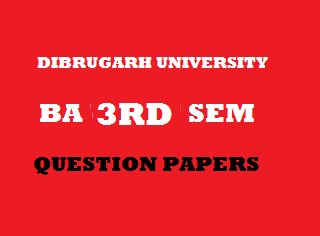## Wednesday, January 02, 20192012
(November)
ECONOMICS
(General)
Course: 301
(Public Economics)
Full Marks: 80
Pass Marks: 32
Time: 3 hours
The figures in the margin indicate full marks for the questions

1. Give the correct answer to the following: 1x8=8
1. In indirect tax
1. Taxpayer bears impact only.
2. Taxpayer bears both impact and incidence of taxes.
3. Taxpayer bears incidence of tax only.
4. Taxpayer bears neither the impact nor the incidence of taxes.
1. Principle of ‘maximum social advantage’ is associated with
1. Wagner.
2. Pigou.
3. Dalton.
4. Taylor.
1. When the rate of tax increases along with the rise in income, it is called
1. Regressive tax.
2. Progressive tax.
3. Proportional tax.
4. None of the above.
1. Which of the following is not administrative revenue?
1. Fees.
3. Fine.
4. Tax.
1. Which of the following is not a method of repayment of public debt?
1. Sinking Fund.
2. Capital Levy.
3. Conversion.
1. In deficit budget
1. Revenue is greater than expenditure.
2. Revenue is smaller than expenditure.
3. Expenditure is equal to revenue.
4. None of the above.

1. One cause of tax evasion is
1. Higher tax rate.
3. Ignorance of taxpayer.
4. All of the above.
1. The Chairman of the 13th Finance Commission is
1. K. C. Neyogi.
2. V. L. Kalkar.
3. Raja Chelliah.
4. C. Rangarajan.
2. Write short notes on any four of the following (within 150 words each): 4x4=16
1. Sources of Government Revenue.
3. Public Goods.
4. Deficit Financing.
5. Indian Finance Commission.
6. Major Recommendations of the 13th Finance Commission.
Answer the following questions (within 500 words each):
3. (a) Define public finance. Discuss the differences between public finance and private finance. 2+9=11
Or
(b) Explain the principle of ‘maximum social advantage’ of public finance. 11
4. (a) What is tax? Explain the objectives of taxation. 2+9=11
Or
(b) Explain, with the help of diagram, the modern theory of incidence of taxation. 11
5. (a) Explain the causes of growing public expenditure in recent years. 11
Or
(b) “Internally held public debt is burden less.” Is the statement correct? Give reasons. 11
6. (a) Explain the procedures followed in the preparation of India’s budget. 11
Or
(b) What is fiscal policy? Explain the objectives of fiscal policy in developing countries. 2+9=11
7. (a) Explain the defects of Indian tax system. Give suggestions to avoid these defects. 8+4=12
Or
(b) Discuss the main characteristics of India’s latest budget for 2012 – 2013. 12

***